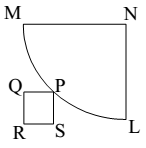In the figure given below MN and NL are adjacent sides of a square and the arc MPL is drawn with N as centre and MN as radius. P is a point on the arc and PQRS is a square such that, RS, if extended, passes through L while RQ, if extended, passes through M. What is the ratio of the area of a square of side MN and the square PQRS?